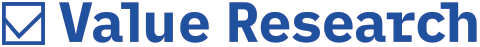# Calculating Annualised Returns

##### I would like to know how one can calculate Annualised returns, especially in cases like SIP and partially realized gains? - Kapil Khanna

In a portfolio, how does one calculate Annualised returns? I would like to know the mathematical formula used, especially in a complex case such as:
1. SIP
2. Partially realized gains
Please try to explain each with an example.
- Kapil Khanna
At Value Research, we use two methods of computing fund returns over a period of time. Returns of fund's performance for a period of less than 1 year are Absolute Returns and that in excess of 1 year are Annualised Returns.

For Annualised returns, we use the CAGR formula i.e. Compound Annualised Growth Rate formula.
If an investment of Rs 5,000, made five years ago has grown to Rs 6,500 today, then the absolute gain would be Rs 1,500 - a 30 per cent growth on initial investment. A 30 per cent return on investment would normally qualify as good but for the fact that it was realized over five years. If you want to know how much the investment has grown on a yearly basis, you will have to take a look at the compounded Annualised growth rate (CAGR).

The CAGR tells you the return a fund turned in every year during the five-year period, provided the gains were re-invested every year. In this case, the CAGR works out to 5.38 per cent. So, in the first year the investment would have grown to Rs 5269. In the second year, it would have been Rs 5552.4 (by adding 5.38 per cent of Rs 5269) and so on. In India, mutual fund regulations require that all returns over one year should be stated in Annualised terms.

On our website, we calculate the returns for less than a year using the absolute method and those above a year as Annualised.